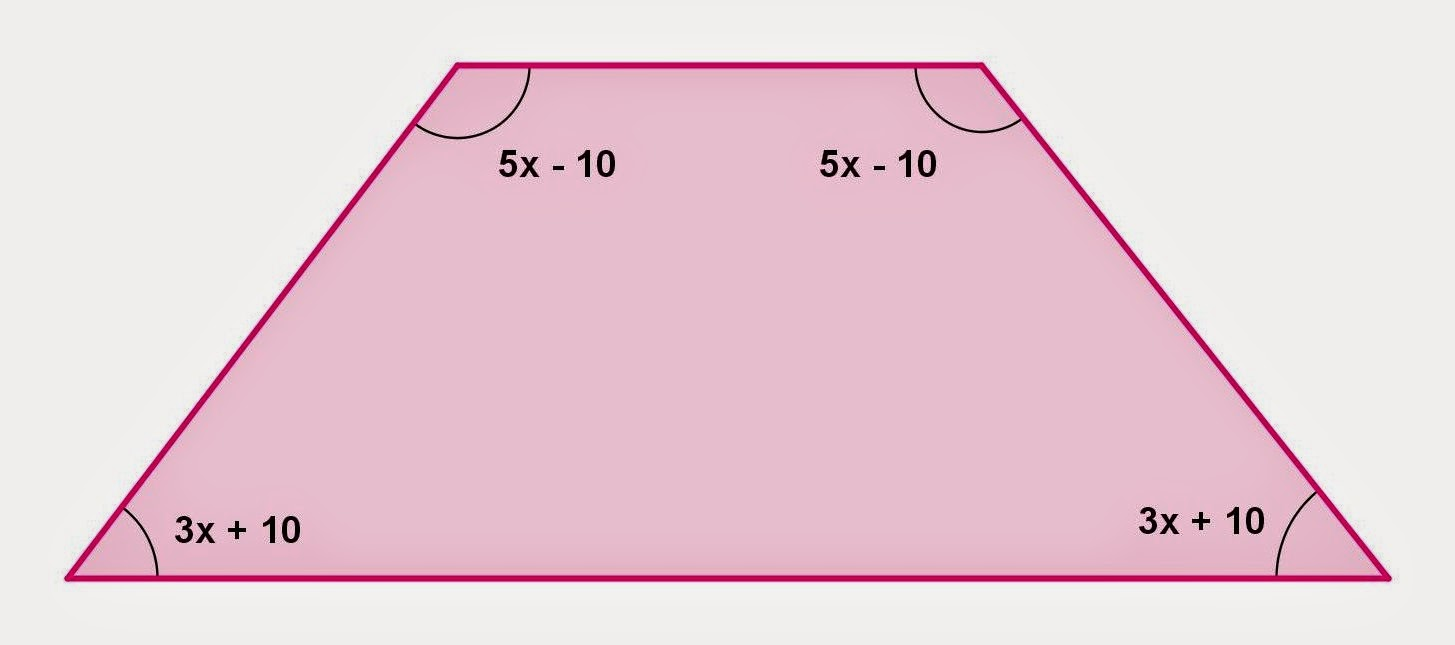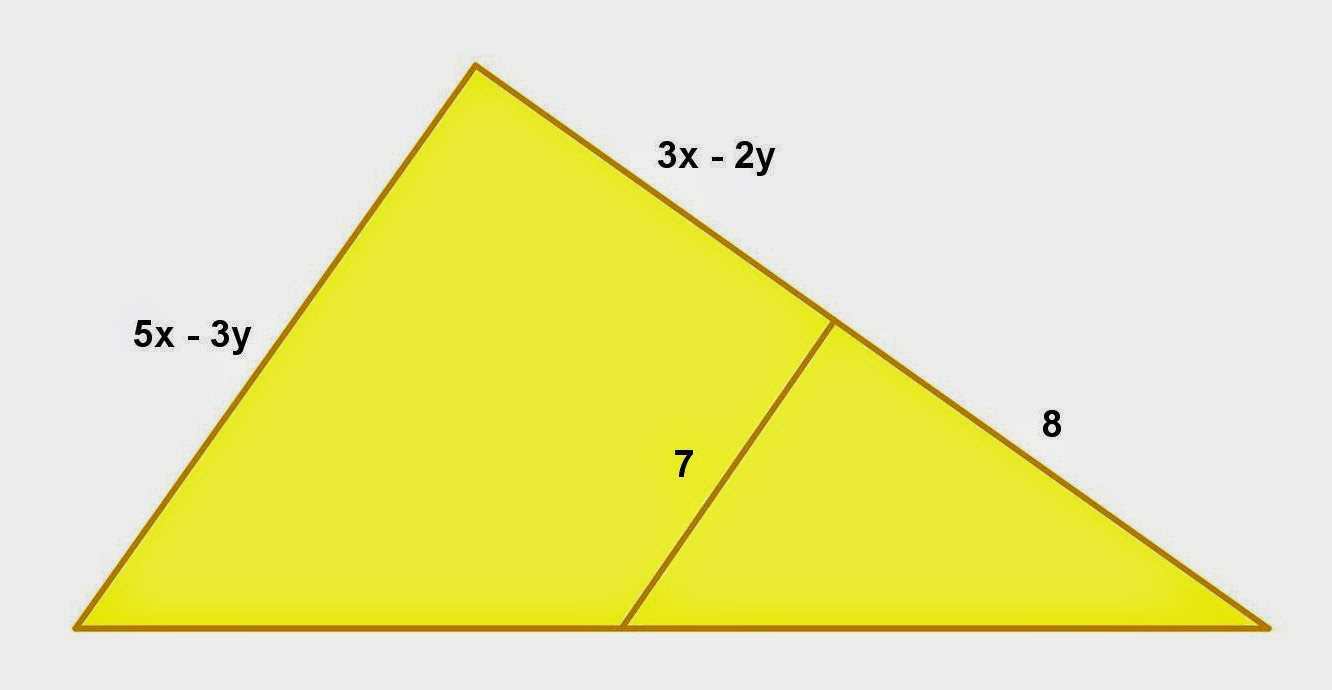Showing posts with label Plane Geometry. Show all posts
Showing posts with label Plane Geometry. Show all posts

## Monday, January 12, 2015

### Trapezoid and Quadrilateral Problems, 7

Category: Plane Geometry

"Published in Newark, California, USA"

Two congruent angles of an isosceles trapezoid have measures 3x + 10 and 5x - 10. Find the value of x and then give the measures of all angles of the trapezoid.

Solution:

To illustrate the problem, it is better to draw the figure as followsPhoto by Math Principles in Everyday Life

Isosceles trapezoid is a trapezoid whose two opposite sides and two adjacent angles are congruent. If the two adjacent angles of the lower base are congruent, then the two adjacent angles of the upper base are also congruent.

For any trapezoid, the two adjacent sides of a leg are supplementary angles because the upper and lower bases are parallel.

If that's the case, the value of x is

Hence, the measurement of an angle at the lower base of an isosceles trapezoid is

and at the upper base is

Therefore, the measurements of the two congruent angles of an isosceles trapezoid are 77.5° and 102.5°.

## Sunday, January 11, 2015

### Trapezoid and Quadrilateral Problems, 6

Category: Plane Geometry

"Published in Newark, California, USA"

One angle of an isosceles trapezoid has measure 57°. Find the measures of the other angles.

Solution:

To illustrate the problem, it is better to draw the figure as followsPhoto by Math Principles in Everyday Life

Isosceles trapezoid is a trapezoid whose two opposite sides and two adjacent angles are congruent. If the two adjacent angles of the lower base are congruent, then the two adjacent angles of the upper base are also congruent.

For any trapezoid, the two adjacent sides of a leg are supplementary angles because the upper and lower bases are parallel.

Therefore, the other angles of an isosceles trapezoid are

There's another way in solving for the other angles of an isosceles trapezoid. For any four closed figures like quadrilateral, trapezoid, parallelogram, rhombus, rectangle, and square, the sum of their internal angles is equal to 360°.

Therefore, the other angles of an isosceles trapezoid are

## Saturday, January 10, 2015

### Trapezoid and Quadrilateral Problems, 5

Category: Plane Geometry

"Published in Newark, California, USA"

Given a trapezoid with its median. Find the value of x.Photo by Math Principles in Everyday Life

Solution:

Consider the given figure abovePhoto by Math Principles in Everyday Life

If a theorem says "The median of a trapezoid is parallel to the bases and has a length equal to the average of the base lengths.", then the value of x, therefore is

## Friday, January 9, 2015

### Trapezoid and Quadrilateral Problems, 4

Category: Plane Geometry

"Published in Newark, California, USA"

Given a trapezoid with its median. Find the value of x.Photo by Math Principles in Everyday Life

Solution:

Consider the given figure abovePhoto by Math Principles in Everyday Life

If a theorem says "The median of a trapezoid is parallel to the bases and has a length equal to the average of the base lengths.", then the value of x, therefore is

## Thursday, January 8, 2015

### Parallel Lines Problems

Category: Plane Geometry

"Published in Newark, California, USA"

Two lines are cut by four parallel lines of equal distance as shown in the figure. Find the values of x and y.Photo by Math Principles in Everyday Life

Solution:

Consider the given figure abovePhoto by Math Principles in Everyday Life

If a theorem says "If three or more parallel lines cut off congruent segments on one transversal, then they cut off congruent segments on every transversal.", then we can equate the two line segments in order to solve for the value of x as follows

Finally, equate the other two line segments in order to solve for the value of y as follows

## Wednesday, January 7, 2015

### Similar Triangles, 4

Category: Plane Geometry

"Published in Newark, California, USA"

In the given figure below, a segment joins the midpoints of two sides of a triangle. Find the values of x and y.Photo by Math Principles in Everyday Life

Solution:

Consider the given figure abovePhoto by Math Principles in Everyday Life

There are two variables in the given figure which are the sides of a big triangle. We need two equations in order to solve for the values of x and y.

Since a line segment joins the midpoints of two sides of a triangle, then the first working equation is

A small triangle is similar to big triangle because a line segment which is its base that joins the midpoints of two sides of a big triangle is parallel to the base of big triangle. By similar triangles, the second working equation is

butTherefore, the value of x is

and the value of y is